# Yield to Worst (YTW)

Understand the Yield to Worst (YTW) Concept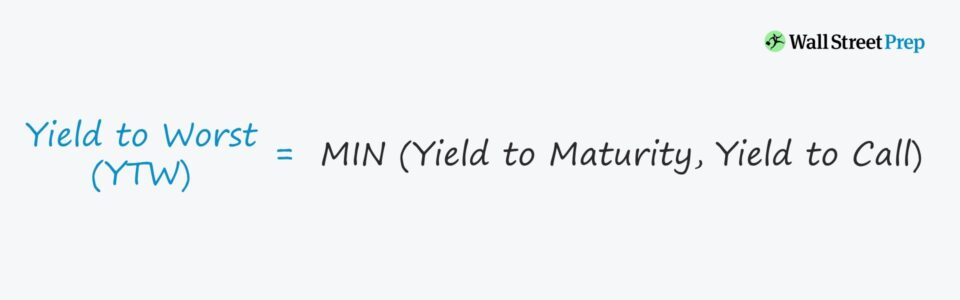• What is the definition of yield to worst (YTW)?
• What does the yield to worst (YTM) on a bond measure?
• Which Excel formula can calculate the yield to worst (YTW)?
• How is the yield to worst (YTW) different from yield to maturity (YTM)?

## Yield to Worst (YTW) Definition

If a bond with a call feature is redeemed at the earliest date without defaulting, then the expected return would be the yield to worst (YTW).

The yield to worst represents the lowest potential yield that a bondholder could receive on a callable bond – assuming the issuer does not default.

Certain types of bond issuances are “callable,” meaning that the issuer has the option to redeem them before the stated maturity date, i.e. pay the debt off earlier.

Within the debentures of such bonds, the earliest date the callable feature is permitted will be clearly stated, along with details regarding any side fees incurred.

As worst-case scenario contingency planning, bondholders often estimate the yield to worst (YTW) – but to clarify, “worst-case” in these cases refer to the bond being redeemed by the issuer at the earliest possible date, rather than the yield on a bond that has defaulted.

From determining the yield to worst (YTW), bondholders can mitigate their downside risk better to reduce the odds of being blindsided by an issuer calling a bond before it matures.

## Yield to Worst (YTW) and Interest Rates

The general rule of thumb is that interest rates and yield have an inverse relationship, i.e. if interest rates rise, bond prices decline (and vice versa).

However, there are more moving pieces to consider for callable bonds, so the prior statement is not necessarily true or false without further context.

More specifically, the issuer could view the low interest rate environment as an opportunity to refinance its existing debt at more favorable rates.

Most bonds are structured with prepayment fees as compensation for the following:

• Reduced Coupon, i.e. Fewer Interest Payments
• Reinvestment Risk

## Yield to Worst (YTW) Importance

In real-life, the yield to worst (YTW) is applicable only for callable bonds and those trading at a premium.

If we assume there are two identical bonds – with the only difference being that one is “callable” whereas the other is “non-callable” – then the callable bond is more likely to be negatively impacted.

Why? The chance of bonds being called tends to increase if interest rates fall and drop lower compared to when the original issuance occurred.

If interest rates decline, the issuer can be incentivized to redeem the bonds, making the callable feature unfavorable for the bondholder.

Calculating the yield to worst (YTW) is most relevant for premium bonds (i.e. “trading above par”), given how they are directly tied to collapsing interest rates.

###### Bond Pricing Review – Discount, Par & Premium
• Discount Bond: YTM > Coupon Rate
• Par Bond: YTM = Coupon Rate
• Premium Bond: YTM < Coupon Rate

## Yield to Worst (YTW) Formula

The yield to worst (YTW) on a callable bond is the lower return between the yield to maturity (YTM) and the yield to call (YTC).

• Yield to Maturity (YTM): The expected internal rate of return (IRR) received on a bond, assuming the bond is held until maturity with coupons reinvested at the same rate.
• Yield to Call (YTC): The anticipated IRR if the bond is redeemed on the earliest call date possible, i.e. the worst-case scenario other than the issuer defaulting.

## Yield to Worst Calculation Steps

In the first step, the yield to maturity (YTM) and yield to call (YTC) can be calculated using the built-in “YIELD” Excel function.

###### YIELD Excel Function
• “= YIELD (settlement, maturity, rate, pr, redemption, frequency)”

The inputs within the formula are as follows:

• settlement = Settlement Date
• maturity = Original Maturity Date or Early Redemption Date
• rate = Annual Coupon Rate
• pr = Bond Quote (% of Par)
• redemption = Par Value or Call Price
• frequency = Number of Compounding Periods (Annual = 1, Semi-Annual = 2)

In the subsequent step, the “MIN” function picks the lower value between the YTM and YTC, i.e. the yield to worst (YTW) of the bond.

###### MIN Excel Function
• “= MIN (Yield to Maturity, Yield to Call)”

## Yield to Worst (YTW) Calculator – Excel Template

We’ll now move to a modeling exercise, which you can access by filling out the form below.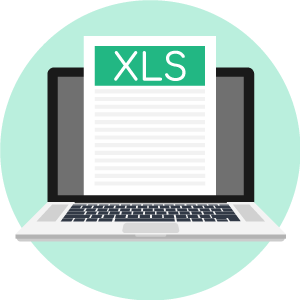Submitting ...

## Yield to Worst (YTW) Example Calculation

In our illustrative exercise, we’ll calculate the yield on the bond under three different pricing scenarios.

Suppose the bond issuance has a maturity of ten years and was finalized on 12/31/2021 with the first call date 12 months after the settlement date.

• Settlement Date: 12/31/21
• Maturity Date: 12/31/31
• First Call Date: 12/31/22 (“NC/1”)

At a par value of \$1,000 (i.e. “100”), the three prices for each scenario are as follows:

1. Discount Bond: \$950 (“95”)
2. Par Bond: \$1,000 (“100”)

As for the coupon, we’ll assume that the bond pays an annual coupon at an interest rate of 6%.

• Frequency of Coupon: 1
• Coupon Rate: 6%
• Annual Coupon: \$60

Now, we’ll enter our assumptions into the Excel formula from earlier to calculate the yield to maturity (YTM):

• Yield to Maturity (YTM): “= YIELD (12/31/2021, 12/31/2031, 6%, Bond Quote, 100, 1)”

By contrast, the YTC switches the “maturity” to the first call date and “redemption” to the call price, which we’ll assume is set at 104.

The call price of 104 is the quoted price the bond issuer must pay to redeem the debt issuance entirely (or partially) before the maturity date.

• Yield to Call (YTC): “= YIELD (12/31/2021, 12/31/2022, 6%, Bond Quote, 104, 1)”

Side Note: From the italics, we can identify which parts of the formula were adjusted in the YTC calculation.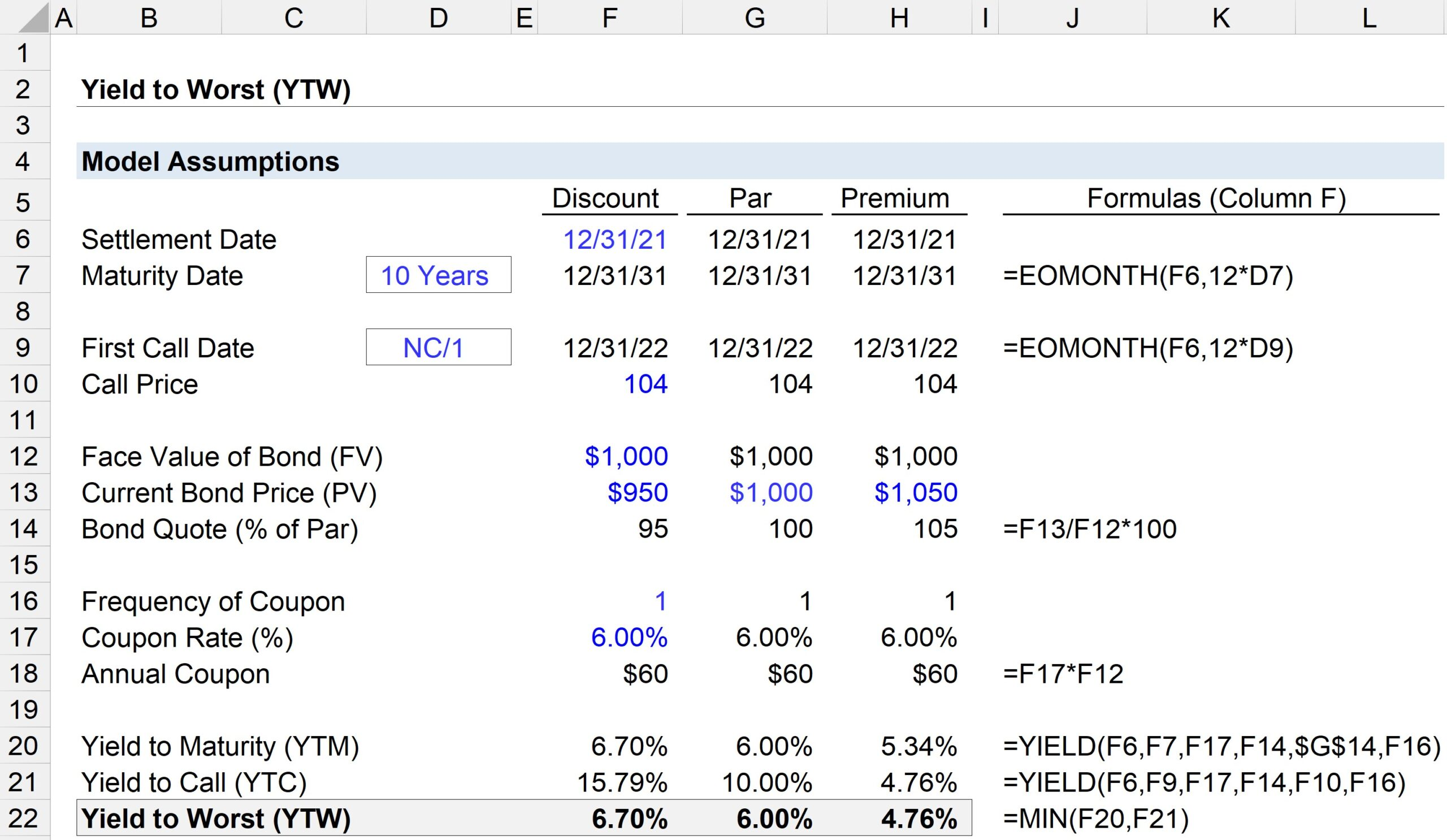If the bond trades at a discount or par, the yield to maturity (YTM) is lower than the yield to call (YTC) – which is why the yield to worst (YTW) is the yield to maturity (YTM).

• Discount Bond: 6.7%% YTM vs 15.8% YTC
• Par Bond: 6.0% YTM vs 10.0% YTC

However, if the bond trades at a premium, the contrary is true, where the YTC is the lower between the two yield metrics and can be considered the YTW.

• Premium Bond: 5.3% YTM vs 4.8% YTC

Unlike the discount and par bond, the premium bond’s yield to worst (YTW) is the yield to call (YTC) of 4.8% – depicting why the YTW is only relevant if the bond is trading at a premium to par.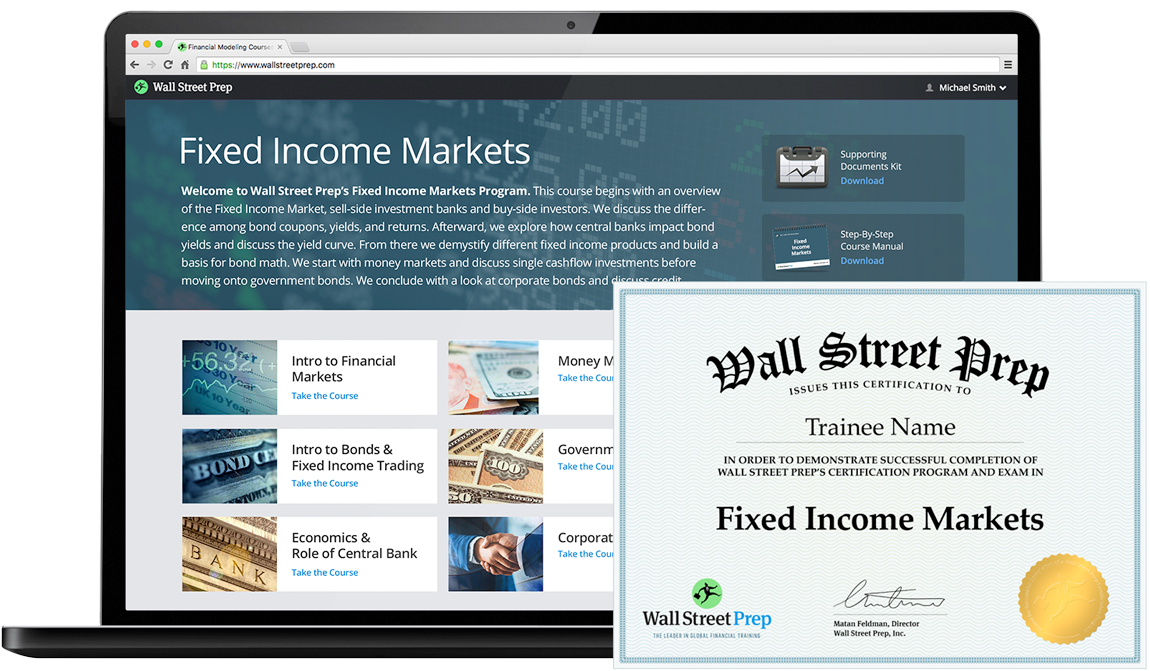Globally Recognized Certification Program

#### Get the Fixed Income Markets Certification (FIMC©)

Wall Street Prep's globally recognized certification program prepares trainees with the skills they need to succeed as a Fixed Income Trader on either the Buy Side or Sell Side.Inline Feedbacks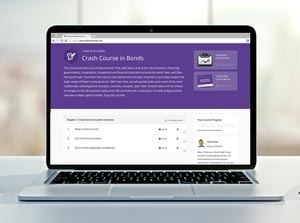Learn Online: Crash Course in Bonds

For those pursuing fixed income research, investments, sales and trading or investment banking.

X

The Wall Street Prep Quicklesson Series

7 Free Financial Modeling Lessons

Get instant access to video lessons taught by experienced investment bankers. Learn financial statement modeling, DCF, M&A, LBO, Comps and Excel shortcuts.